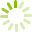# Discrete Mathematics 1 (MATH 1120)

This course introduces students to mathematical logic, mathematical induction, relations and functions, basic counting techniques, probability, graphs and trees, with an emphasis on applications in computer science.
Course code: MATH 1120
Credits: 3.0
Length: 60.0 hours
Course outline: view https://www.vcc.ca/vccphp/courseoutline?subject=MATH&number=1120

## Prerequisites

Precalculus 12 with a minimum grade of 'C' or MATH 1020 with a minimum grade of 'C' or VCC Math Precalculus Assessment Test (MPT) with a minimum 72%.